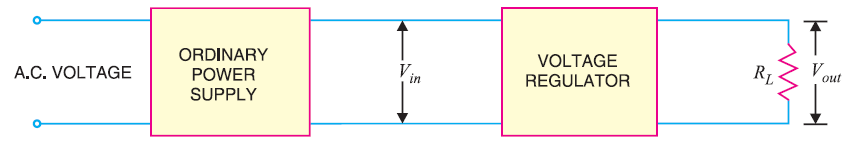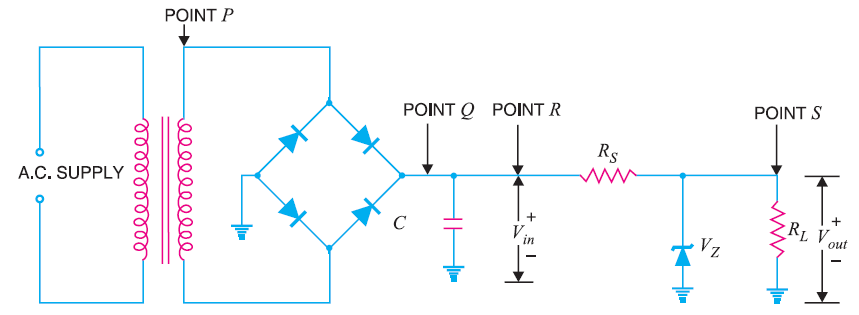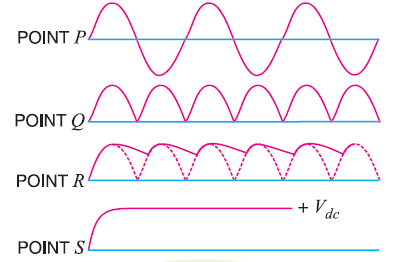# Regulated DC Power Supply

## Regulated DC Power Supply

A d.c. power supply which maintains the output voltage constant irrespective of a.c. mains fluctuations or load variations is known as regulated dc power supply .

For a regulated power supply you will need an ordinary power supply and voltage regulating device. The figure below shows the block diagram of a regulated power supply .Here we feed the output of ordinary power supply to the voltage regulator which in turn produces the final output. The output voltage remains constant despite changes in the load current changes or fluctuations in the input a.c. voltage.

In the figure below you can see the circuit diagram of a regulated power supply where zener diode is used as a voltage regulating device.The regulated power supply consists of three circuits :

• Bridge rectifier ,
• Capacitor filter C , &
• Zener voltage regulator.

The purpose of the bridge rectifier is to convert the transformer secondary a.c. voltage (point P) into pulsating voltage (point Q). Again this pulsating d.c. voltage is applied to the capacitor filter.

The purpose of the capacitor filter is to reduce the pulsations in the rectifier d.c. output voltage (point R).

Thus main functions of the zener voltage regulator are :

Reducing the variations in the filtered output voltage.

Keeping the output voltage (Vout) almost constant despite changes in the load current or input a.c. voltage.

To see the waveforms at various stages of a regulated power supply see the figure below :### What Is The Need of Regulated Power Supply

In an unregulated power supply, that is, under ordinary power supply conditions, the voltage regulation is not good. Changes in the load current cause the output voltage to change as well. Again ,the variations in the input a.c. voltage causes the output voltage to change as well .

This happens due to the following reasons :

• Under practical situations, there are a lot of factors , there are a lot of variations in a.c. line voltage that are not in our control. This causes the d.c. output voltage to fluctuate. Most of the electronic circuits wouldn’t work properly on such output voltage fluctuations. Hence we need a regulated dc power supply .
• The internal resistance of ordinary power supply is relatively large (> 30 Ù). This in turn affect the load current drawn from the supply. These variations in d.c. voltage may result in the erratic operation of electronic circuits. Hence we need a regulated dc power supply .

You Might Like The Following Articles